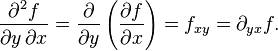# Notation for 2nd-order mixed derivatives

## Homework Statement

According to Wikipedia and one of my calculus textbooks: (I am used to this notation)But I've seen another source writing fxy=∂2f/∂x∂y=∂xyf which is totally inconsistent with the above. How come?

Which one is standard? Is the second one wrong, or is it acceptable? The second one is driving me crazy...is it ever used anywhere?

N/A

## The Attempt at a Solution

N/A

Thank you!

Dick
Homework Helper
If you replace f_xy in your first example with f_yx, then I'm with you. For basically all examples of interest in physics or the sciences, f_xy=f_yx. Hence, the free interchange of x and y. In mathematics there are examples of functions where f_xy is not equal to f_yx.

If you replace f_xy in your first example with f_yx, then I'm with you. For basically all examples of interest in physics or the sciences, f_xy=f_yx. Hence, the free interchange of x and y. In mathematics there are examples of functions where f_xy is not equal to f_yx.

fxy means (fx)y, no?
So I would interpret fxy as taking partial derivative w.r.t. x first and then w.r.t. y which is consistent with my calculus textbook.

However, in my studies of partial differential equations (which I would classify as math rather than science), they write fxy=∂2f/∂x∂y=∂xyf which is inconsistent with the above.
2f/∂x∂y means taking partial derivative w.r.t. y first and then w.r.t. x, but fxy to me is the opposite.

Which notation is standard in mathematics?

Thanks!

Last edited:
Dick
Homework Helper
You know, I've never thought about it that hard. Wikipedia says ∂2f/∂x∂y is f_yx. I guess I'll trust them. The operator notation is clearer.

D H
Staff Emeritus
Which one is standard? Is the second one wrong, or is it acceptable? The second one is driving me crazy...is it ever used anywhere?
http://mathworld.wolfram.com/MixedPartialDerivative.html, for one.

What is the "correct" way to denote a similarity transformation:
$$QAQ^{-1}$$ or $$Q^{-1}AQ$$

What is the "correct" way to represent a rotation by angle $\theta$ about an axis $\hat u$ as a quaternion:
$$\bmatrix \cos \theta/2 \\ \hat u \sin \theta/2\endbmatrix$$ or $$\bmatrix \cos \theta/2 \\ -\hat u \sin \theta/2\endbmatrix$$ or $$\bmatrix\hat u \sin \theta/2 \\ \cos \theta/2 \endbmatrix$$ or $$\bmatrix -\hat u \sin \theta/2 \\ \cos \theta/2 \endbmatrix$$

What is the "correct" way to represent $\partial/\partial y\left(\partial f/\partial x\right)$:
$$f_{xy}[/itex] or [tex]f_{yx}$$

The correct answer is: Asking which way is the "correct" way is wrong. There are lots of cases where different nomenclatures are used in different places. I just gave three examples; there are many more. You need to recognize that sometimes there are multiple ways to write things. Sometimes the chosen nomenclature is obvious from context. Sometimes it isn't so obvious; hopefully the author of a paper describes the nomenclature. Sometimes not. Such is life.

Last edited: